International
Tables for
Crystallography
Volume A
Space-group symmetry
Edited by M. I. Aroyo

International Tables for Crystallography (2016). Vol. A, ch. 1.1, pp. 4-5

Section 1.1.3. Subgroups

B. Souvigniera*

aRadboud University Nijmegen, Faculty of Science, Mathematics and Computing Science, Institute for Mathematics, Astrophysics and Particle Physics, Postbus 9010, 6500 GL Nijmegen, The Netherlands
Correspondence e-mail: souvi@math.ru.nl

1.1.3. Subgroups

| top | pdf |

The group of symmetry operations of a crystal pattern may alter if the crystal undergoes a phase transition. Often, some symmetries are preserved, while others are lost, i.e. symmetry breaking takes place. The symmetry operations that are preserved form a subset of the original symmetry group which is itself a group. This gives rise to the concept of a subgroup.

Definition. A subset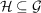is called a subgroup ofif its elements form a group by themselves. This is denoted by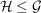. Ifis a subgroup of, thenis called a supergroup of. In order to be a subgroup,is required to contain the identity elementof, to contain inverse elements and to be closed with respect to composition of elements. Thus, technically, every group is a subgroup of itself.

The subgroups ofthat are not equal toare called proper subgroups of. A proper subgroupofis called a maximal subgroup if it is not a proper subgroup of any proper subgroupof.

It is often convenient to specify a subgroupofby a set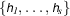of generators. This is denoted by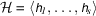. The order ofis not a priori obvious from the set of generators. For example, in the symmetry group 4mm of the square the pairs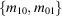and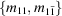both generate subgroups of order 4, whereas the pair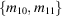generates the full group of order 8.

The subgroups of a group can be visualized in a subgroup diagram. In such a diagram the subgroups are arranged with subgroups of higher order above subgroups of lower order. Two subgroups are connected by a line if one is a maximal subgroup of the other. By following downward paths in this diagram, all group–subgroup relations in a group can be derived. Additional information is provided by connecting subgroups of the same order by a horizontal line if they are conjugate (see Section 1.1.7).

Examples

• (i) The set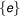consisting only of the identity element ofis a subgroup, called the trivial subgroup of.

• (ii) For the group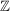of the integers, all subgroups are cyclic and generated by some integer n, i.e. they are of the form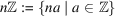for an integer n. Such a subgroup is maximal if n is a prime number.

• (iii) For every element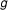of a group, the powers ofform a subgroup ofwhich is a cyclic group.

• (iv) In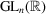the matrices of determinant 1 form a subgroup, since the determinant of the matrix product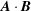is equal to the product of the determinants of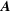and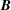.

• (v) In the symmetry group 3m of an equilateral triangle the rotations form a subgroup of order 3 (see Fig. 1.1.3.1).Figure 1.1.3.1 | top | pdf |Subgroup diagram for the symmetry group 3m of an equilateral triangle.
• (vi) The symmetry group 2mm of a rectangle has three subgroups of order 2, generated by the reflection m10, the twofold rotation 2 and the reflection m01, respectively (see Fig. 1.1.3.2).Figure 1.1.3.2 | top | pdf |Subgroup diagram for the symmetry group 2mm of a rectangle.
• (vii) In the symmetry group 4mm of the square, the reflections m10 and m01 together with their product 2 and the identity element 1 form a subgroup of order 4. This subgroup can be recognized in the subgroup diagram of 4mm as the subdiagram of the subgroups of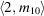in the left part of Fig. 1.1.3.3which coincides with the subgroup diagram of 2mm in Fig. 1.1.3.2. A different subgroup of order 4 is formed by the other pair of perpendicular reflections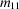,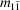together with 2 and 1 and a third subgroup of order 4 is the cyclic subgroup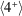generated by the fourfold rotation (see Fig. 1.1.3.3).Figure 1.1.3.3 | top | pdf |Subgroup diagram for the symmetry group 4mm of the square.